# Test: Fluid Pressure & Measurement

## 10 Questions MCQ Test Topicwise Question Bank for Civil Engineering | Test: Fluid Pressure & Measurement

Description
Attempt Test: Fluid Pressure & Measurement | 10 questions in 30 minutes | Mock test for Civil Engineering (CE) preparation | Free important questions MCQ to study Topicwise Question Bank for Civil Engineering for Civil Engineering (CE) Exam | Download free PDF with solutions
QUESTION: 1

Solution:
QUESTION: 2

### In a mercury differential manometer used for measuring pressure differences across a venturimeter in a water pipeline, if an error of 2 mm has been made in observing a differential head of 10 mm, the percentage error in pressure difference is:

Solution: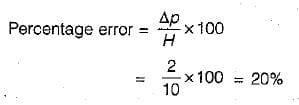QUESTION: 3

### What is the pressure in Pascals at a depth of 1m below the water surface?

Solution:

It’s the summation of weights on top of the water surface. In this case, it is the weight of the atmosphere and water above 1m. [Formula: P (depth) = Patm + (density of water*gravitational constant * depth)].

QUESTION: 4

The left leg of a U-tube mercury manometer is connected to a pipeline conveying water, the level of mercury in the leg being 0.6 m below the centre of pipeline, and the right leg is open to atmosphere. The level of mercury in the right leg is 0.45 m above that in the left leg and the space above mercury in the right leg contains Benzene (specific gravity 0.88) to a height of 0.3 m. Find the pressure in the pipe in metres of water.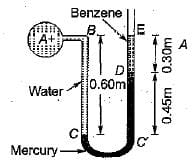Solution: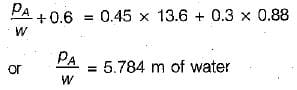QUESTION: 5

The pressure, in meters of oil (sp. gr, 0,8), equivalent to 80 m of water is

Solution: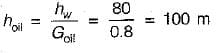QUESTION: 6

Match List-1 (Features) with List-ll .(Type of manometers) and select the correct answer using the codes given below the lists:
List-I
A. Open ended manometer for positive pressure
B. Negative pressure manometer
C. For measuring pressure in liquids or gases
D. For measuring pressure in liquids only

List-ll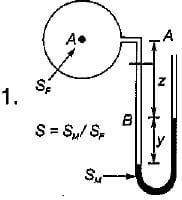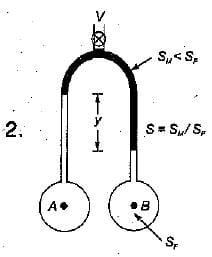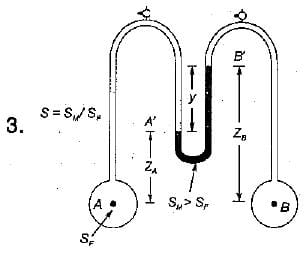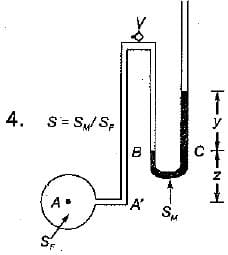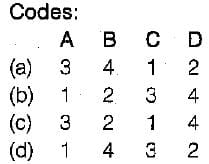Solution:
QUESTION: 7

Absolute pressure in flow system

Solution:

The pressure measured above the absolute zero or complete vacuum is called absolute pressure.
Absolute pressure = Gauge pressure + Local atmosphere pressure.
also Absolute pressure = Local atmosphere pressure - Negative gauge pressure.

QUESTION: 8

Pressure of 200 kPa is equivalent to a head of h metres of carbon tetrachloride of relative density 1.59 where h is equal to

Solution: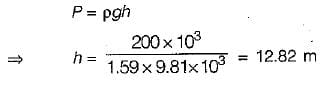QUESTION: 9

Mercury is considered as a superior barometric fluid chiefly due to its

Solution:

Mercury is considered as a superior barometric fluid because of following reasons:
1. High specific gravity- For a same pressure head mercury tube length required will be smaller than that of water
2.Low Vapour Pressure: Thus, influence in readings due to mercury vapours is negligible.

QUESTION: 10

If a Mohr's circle is drawn for a fluid element inside a fluid body at rest, it would be

Solution:Use Code STAYHOME200 and get INR 200 additional OFF Use Coupon Code